﻿ The Use of Finite Element Method in the Stress Analysis of an Industrial Operation Machine

### The Use of Finite Element Method in the Stress Analysis of an Industrial Operation MachineOPEN ACCESSPEER-REVIEWED

## The Use of Finite Element Method in the Stress Analysis of an Industrial Operation Machine

Ladislav NovotnýDepartment of Applied Mechanics and Mechatronics, Technical University of Košice, Košice, Slovakia

### Abstract

This paper presents an example of a stress analysis of the arm of an industrial operation machine. The introduction describes the systematic design and stress calculation of the constituent parts of the industrial operation machine. For stress analysis, the finite element method was chosen, whereby a brief description of the method and the finite elements is given. The paper describes the creation of the finite element model of the arm of the industrial operation machine, together with the courses of the resulting final deformation and displacement, as well as the resulting von Misses stress behaviour.

### At a glance: Figures

123
Prev Next

• Novotný, Ladislav. "The Use of Finite Element Method in the Stress Analysis of an Industrial Operation Machine." American Journal of Mechanical Engineering 2.7 (2014): 184-187.
• Novotný, L. (2014). The Use of Finite Element Method in the Stress Analysis of an Industrial Operation Machine. American Journal of Mechanical Engineering, 2(7), 184-187.
• Novotný, Ladislav. "The Use of Finite Element Method in the Stress Analysis of an Industrial Operation Machine." American Journal of Mechanical Engineering 2, no. 7 (2014): 184-187.

 Import into BibTeX Import into EndNote Import into RefMan Import into RefWorks

### 1. Introduction

Different types of industrial operation machines differ from the design point of view, because they are developed for a different character of loads and methods of manipulation, multiple operating conditions, different types of load weights, etc. The variability of the industrial operation machine is important especially when inserting it between two continuously operating devices. A very important technical and economic factor is the speed and economic efficiency of the production of the industrial operation machine, which can rarely be achieved by using standardized components.

Figure 1. The industrial operation machine designed to work with metal coils

The subject of the stress analysis is the arm of the industrial operation machine shown in Figure 1. The aim of the industrial operation machine is to change the position of a sheet metal coil from vertical to horizontal and its subsequent transfer by a roller conveyor. In the horizontal position of the industrial operation machine’s arm, it is possible to place a sheet metal coil on the arm, the arm rotation changes the sheet metal coil position from horizontal to vertical, and a subsequent transfer of the sheet metal coil by the roller conveyor would be possible.

### 2. The Description and Development Process of the Industrial Operation Machine

The industrial operation machine shown in Figure 1 consists of a carrier construction, which forms the carrier system of the industrial operation machine, and gives it an external contour.

The carrier construction is made of construction steel with the yield stress of.

A mechanism which provides a working movement of the industrial operation machine is placed inside of the steel construction.

The mechanism is made and moved by a hydraulic cylinder. The mechanism design itself is created by a functional design of the mechanism, and by a strength calculation of its part.

The functional calculation consists of a calculation of power parameters of the propulsion system, i.e. the power of a driven aggregate in the chosen tilting mode of the sheet metal coils, and the kinematics values of individual mechanism constituents.

The strength calculation of the industrial operating machine’s arm is described in detail in the following part.

2.1. Stress Analysis of the Operating Machine’s Arm

The strength calculation was performed in two stages. In the first stage, the main parameters of the operating machine’s arm parts were designed on the basis of preliminary strength calculations, and approximate and simpler, single calculation methods were used.

The model of the operating machine’s arm design is presented in Figure 2 (CAD model). The arm is designed as a weld of steel sheet parts.

2.1.1. Finite Components Method Stress Analysis

There are 15 equations (6 geometrical equations, 3 differential equilibrium equations, 6 constitutional equations) to find 15 searched functions (3 displacement functions, 6 functions of the component of strain tensor, 6 functions of the component of stress tensor) in continuum mechanics. Moreover, boundary conditions, namely geometrical, that determines the displacement values on the parts of the body borders and force boundary conditions that determine the stress and pressure values on another part of the body, have to be satisfied. The analytical solution of these equations is possible only for limited simple body shape designs. For more complex body designs appearing in a real engineering practice, these equations are solved by numerical methods, mostly by using the finite element method. If it is used, the researched part is divided into small parts, the so called finite elements. Within the scope of individual finite element, searched functions are interpolated by base functions(1)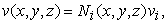(2)(3)

whereas these base functions are selected as applicable as follows(4)(5)

In equations (4) and (5),are coordinates of node number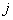.

During the stress analysis of the operating machine’s arm, linear 8-node volume elements - hexahedrons and tetrahedrons were used (Figure 5). The 8-node volume finite element have the interpolation functions defined by the relation(5)

For example, the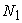function, where we put coordinates of the node 1 (,,) is defined by the relation(6)

For the coordinates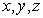of a real finite element displayed in Figure 4, these transformation equations are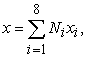(7)(8)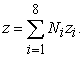(9)

When using tetrahedrons elements (Figure 5), the interpolations functions of a 4-node element are chosen in the following form(10)

whereas,,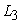,are volume coordinates defined by the relations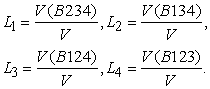(11)

From the geometrical equations point of view (assumption of small deformations), thus it is true that(12)(13)(14)(15)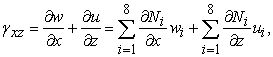(16)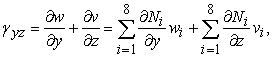(17)

With this, we achieve that we derive known interpolation functions instead of partial derivatives of unknown functions, and only the displacement of element node is searched for.

When calculating node displacements, we count on the principle of virtual work, or in case of a linear elastic material from the principle of the minimum total potential energy. From the principle of virtual work defined by the relation(18)

applies that, external virtual work is equal to internal virtual work when equilibrated forces and stresses undergo unrelated but consistent displacements and strains.

2.1.2. The FEA Application in the Stress Analysis of an Operating Device Arm

Though, the model is symmetrical with reference to one plane, the symmetry was not used because of the following calculation of the frequencies themselves and buckling calculations  that are not contained in this article . The boundary conditions were prescribed at the place of the swivel pins, and in the pin mounting of the hydraulic cylinder.

The loading corresponding to the double scroll weight of the sheet coil, was applied by the form of pressure having effect on the upper panel.

The whole arm is made of the St430C material (steel class EN 10025 S275JO). Maximum of calculated von Misses stress can by(19)

whereas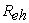is the yield stress,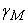is the coefficient of material reliability .

When calculating, the material was considered as isotropic and linear elastic, with elastic constants: Young module, Poisson ratio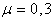.

2.1.3. Interpretation of the Simulation Results

Values of the Von Misses stress are displayed in Figure 7 and in Figure 8.

The maximal stress value is. The behaviour of Von Misses stress on the back part of the operating machine’s arm is presented in Figure 8.

From the functional point of view, it is necessary not to have large deformations of the operation machines arm, because it would cause the downslide of the sheet metal coil from the arm .

In Figure 9, there is portrayed the distribution of the arm resultant displacements. The largest displacement is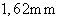at the place of free end of the arm.

### 3. Conclusion

In this paper, an example of stress analysis using finite element method of a in an industrial operating machine has been described. The method principle as well as the solution results have been provided. On the basis of the calculated stress and values of displacement, we can assert that calculated values of Von Misses stress did not surpass the value of the allowed stress. Moreover, the extent of the operating machine’s arm deformation is not that big as to prevent the correct functioning and operation of this constituent.

### Acknowledgement

This research was supported by the Scientific Grant Agency of the Ministry of Education of Slovak Republic under grant no. 2/0098/14.

### References

  Ivančo, V., Vodička, R., The Numerical Methods of the Mechanics of Elements and Selected Applications, Košice, 2012.In article  Santafé Iribarren, B., Berke, P., Bouillard, PH., Vantomme, J., Massart, T.J., Investigation of the influence of design and material parameters in the progressive collapse analysis of RC structures. Engineering Structures, v. 33, 2011, 2805-2820.In article CrossRef  Semrád, K., Fatigue and Buckling Strength Analysis of the Wing Hinge in the Creo Simulation Environment, Journal of Mechanics Engineering and Automation. Vol. 3, no. 4, 2013, 247-250.In article  STN 731401. Design of Steel Structures. Slovak Institute for Technical Standardization. Bratislava 1998.In article  Vega, A., Rashed, S., Tango, Y., Ishiyama, M., Murakawa, H., Analysis and prediction of multiheating lines effect on plate forming by line heating. CMES Journal: Computer Modeling in Engineering & Sciences, CMES, Vol. 28, No. 1, 2008., 1-14.In article